Published: 18 September 2015

# Frequency domain analysis of multiple modules-offshore mobile platform

H. X. Li1
Y. M. Chen2
J. K. Liu3
Z. W. Wu4
1, 2, 3, 4Department of Mechanics, Sun Yat-sen University, Guangzhou 510006, P. R. China
Corresponding Author:
Y. M. Chen
Views 29

#### Abstract

Multiple modules-offshore platform is a typical dynamic network with ﬂexible-rigid-ﬂuid coupling. The determination of the motions of this system in response to environmental forces and structure control mechanisms is a complex procedure, which may include system nonlinearities and position-dependent environmental loads, as well as any motion control mechanisms. For a systematic parametric study, given the large number of possible combinations of environmental conditions, a time domain analysis would be prohibitively time-consuming. However, frequency domain evaluation of AQWA can provide a simple and fast tool to fulfill this requirement.

## 1. Introduction

Multiple modules-offshore mobile platforms can be a sea comprehensive supply base with refueling, aircraft movements, logistics and other functions. More importantly, it can be used to protect national maritime rights. Therefore, it set off research upsurge of very large floating structures in international ocean engineering. Scholars from all over the world have spread a lot of theories of very large floating ocean structure [1-3]. Japanese scholars study pontoon offshore platforms and test the feasibility of floating platform [4-7]. Compared with Japan, the United States put forward the semi-submersible offshore base and related research [8-9]. Xu Daolin  set up a rigid-soft coupling network system. But it is only analyzed the one-dimensional network mechanics system composed of single direction connection. This paper will be based on the AQWA to analyze two-dimensional floating network system. This work provides a new methodology and an application example in the study for network structural dynamics, including very large scale ﬂoating structures.

## 2. Theory and models

### 2.1. Response spectral density

In a linear dynamic system consisting of $N$ structures, the equation of motion in the frequency domain is written as:

1
$\left[-{\omega }^{2}M-i\omega C+K\right]U=F,$

where $M$, $C$ and $K$ are the 6$N$×6$N$ mass, damping, and stiffness matrices respectively, $U$ is the 6$N$×1 motion response, and $F$ is the 6$N$×1 external force, at frequency $\omega$.

In Eq. (1), $\left[-{\omega }^{2}M-i\omega C+K\right]$ is called the impedance matrix, while the receptance matrix is defined as:

2
$H={\left[-{\omega }^{2}M-i\omega C+K\right]}^{-1}.$

The motion response in complex values can then be expressed as:

3
$U=HF.$

In multi-directional waves, denoting the ordinate of the $m$th directional wave spectrum in direction ${\chi }_{m}$ at frequency $\omega$ as ${S}_{\omega m}$, the 6$N$×6$N$ general transform function due to the first order wave ex-citation is defined as:

4
$T=\sum _{m=1}^{{N}_{d}}{S}_{\omega m}\left(\omega \right){U}^{*}\left(\omega ,{\chi }_{m}\right){U}^{T}\left(\omega ,{\chi }_{m}\right),$

where the superscripts ‘$*$’ and $T$ indicate the conjugate transpose and non-conjugate transpose of a matrix respectively, and ${N}_{d}$ is the number of wave directions. The diagonal terms of the real part of the general transform function matrix are the motion response spectral densities, i.e.

5
${S}_{{U}_{j}}\left(\omega \right)=\sum _{m=1}^{{N}_{d}}{S}_{\omega m}\left(\omega \right){\left|{U}_{j}\left(\omega ,{\chi }_{m}\right)\right|}^{2},\mathrm{}j=1,\mathrm{}6,\dots ,\mathrm{}N.$

Wave excitation force spectral density is:

6
${\mathrm{S}}_{{F}_{j}}\left(\omega \right)=\sum _{m=1}^{{N}_{d}}{\mathrm{}\mathrm{S}}_{\omega m}\left(\omega \right){\left|{F}_{j}\left(\omega ,{\mathrm{\chi }}_{m}\right)\right|}^{2}.$

### 2.2. Geometry and environmental parameters

Multiple modules-offshore mobile platform is composed of six same models through cable and Fender, as shown in Fig. 1. Denoting $k$ as the mooring line stiffness and ${L}_{0}$ as its initial unstretched length, and ${X}_{1}\left(t\right)$, ${X}_{2}\left(t\right)$ as the attachment points on the two structures (in the fixed reference axes, where one structure may be a fixed location, for instance an anchor point), the tension on the mooring line is defined as:

7
$T=\left\{\begin{array}{ll}k\left(L-{L}_{0}\right),& L>{L}_{0},\\ 0,& L\le {L}_{0},\end{array}\right\$

where the stretched length of the cable is $L=\left|{X}_{1}\left(t\right)-{X}_{2}\left(t\right)\right|$.

Fig. 1Multiple modules-offshore mobile platform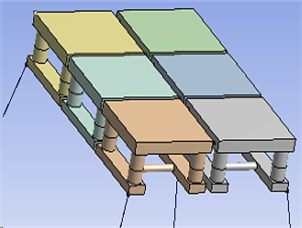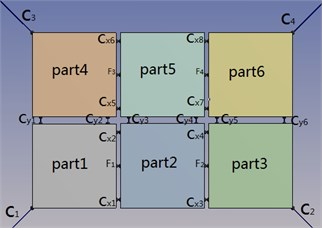The magnitude of the fender axis-directional compression force is defined as a polynomial function of the compression, as:

8
$T=\left\{\begin{array}{ll}{k}_{1}∆L+{k}_{2}{{\left(∆L\right)}^{2}+k}_{3}{\left(∆L\right)}^{3}{+k}_{4}{\left(∆L\right)}^{4}+{k}_{5}{\left(∆L\right)}^{5},& ∆L>0,\\ 0,& ∆L\le 0,\end{array}\right\$

where ${k}_{j}$ ($j=$ 1, 5) are the coefficients of the polynomial function and $∆L={L}_{0}-L$.

The main characteristic parameters of a single module is shown in Table 1. This paper adopts a once-in-a-century extreme condition in South China Sea, as shown in Table 2.

Table 1The main characteristic parameters of a single module

 Parts Parameters size Deck (length $X$ width $X$ height) (m) 100×100×14 Pillar (high $X$ diameter) (m) 35×17 Buoy (length $X$ width $X$ height) (m) 100×18×10 Beam pillar (diameter) (m) 8 Other parameters Normal waterline 25 m Displacement46440e3 m3 ${I}_{xx}=$ 7.91e10 (kg∙m2) ${I}_{yy}=$ 6.49e10 (kg∙m2) ${I}_{zz}=$ 9.35e10(kg∙m2) Center of gravity from the waterline 5.0 m

 Parameters The numerical The waves Significant wave height /m 13.5 Peak period/s 15.5 Angle / (°) 180 or 225

## 3. Frequency domain analysis

Due to large amounts of data, the paper selectedpart1 constrained by the anchor and part5 located in the central position of the chain structure to analyze. There is little literature research double row floating structure system, so this paper focuses on the lateral cables ${C}_{y1}$-${C}_{y6}$, study how the two floating bodiescoupling in the lateral.

Fig. 2The amplitude of horizontal swing and the heave for part1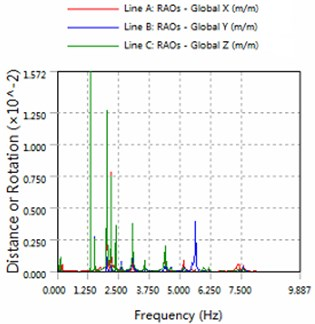a) 180 degree angle for wave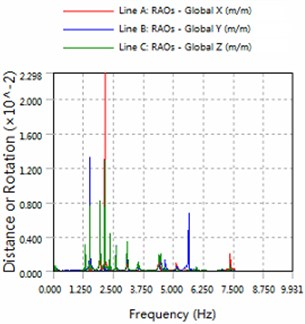b) 225 degree angle for wave

By analyzing Figs. 1 to 7, can be obtained summary:

1) In the same sea conditions, part5 motion response amplitude is greater than part1. Because part1 constraints by the anchor, so it is realistic.

2) The platform is sensitive to the wave frequency of 1.25 Hz-7.5 Hz range. In engineering application, we prevent the frequency of this region to damage multiple modules-offshore mobile platform.

3) The magnitude of floating structure and cable and fender at wave of 180 degree angle are less than the magnitude at wave of 225 degrees. Therefore, in engineering applications, modules-offshore mobile platform should give more consideration to against the waves to arrange.

Fig. 3The amplitude of roll, pitch and the yaw for part1a) 180 degree angle for wave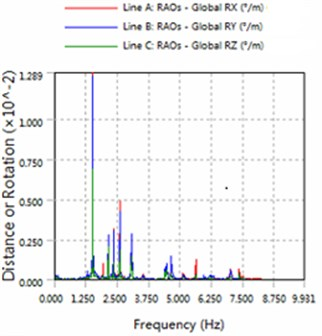b) 225 degree angle for wave

Fig. 4The amplitude of horizontal swing and the heave for part5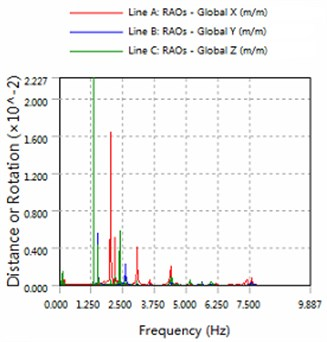a) 180 degree angle for wave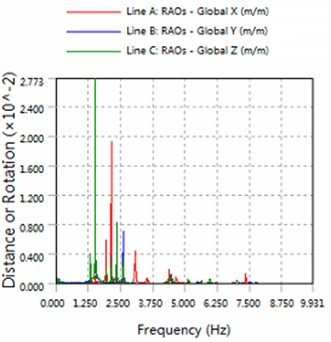b) 225 degree angle for wave

Fig. 5The amplitude of roll, pitch and the yaw for part5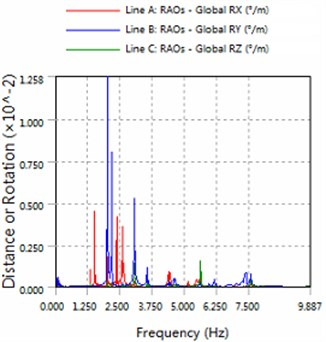a) 180 degree angle for wave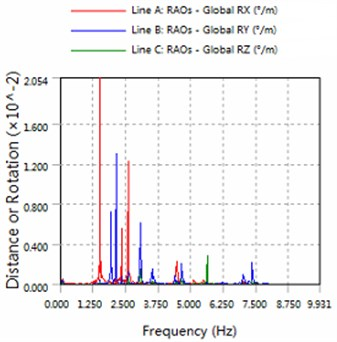b) 225 degree angle for wave

Fig. 6The amplitude of cable force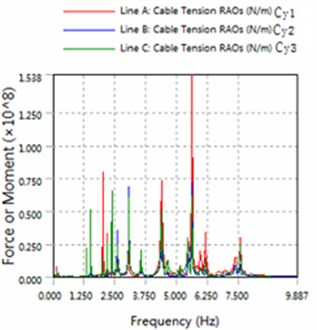a) 180 degree angle for wave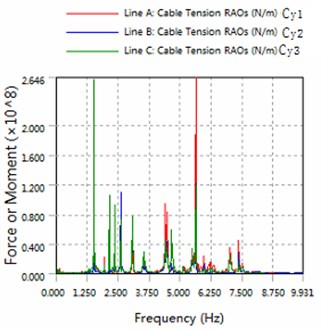b) 225 degree angle for wave

Fig. 7The amplitude of fender force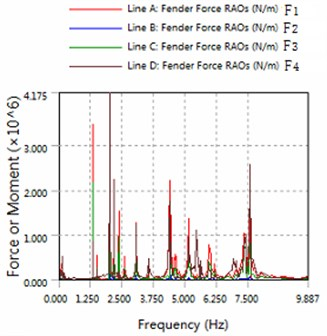a) 180 degree angle for wave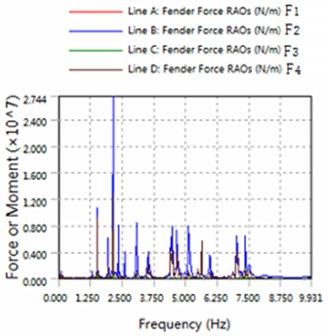b) 225 degree angle for wave

## 4. Conclusions

At present few literature study multi-row and multiple modules-offshore platform, this paper use AQWA for frequency domain analysis of this platform. And preliminary results are obtained. Frequency domain analysis can get exact solution quickly and can provide the reference that whether designers decided to improve the system. This work provides a new methodology and an application example in the study for network structural dynamics, including very large scale ﬂoating structures.

Accepted
31 August 2015
Published
18 September 2015
SUBJECTS
Modal analysis
Keywords
modules-offshore mobile platform
network dynamics
frequency domain analysis
AQWA
Acknowledgements

This work is supported by the National Natural Science Foundation of China (11572356, 111272361), Doctoral Program Foundation of Ministry of Education of China (20130171110039), Guangdong Province Natural Science Foundation (1414050000412, S2013010013802), the Guangdong Province Science and Technology Program (2012A030200011), and Fundamental Research Funds for the Central Universities (15lgzd01).